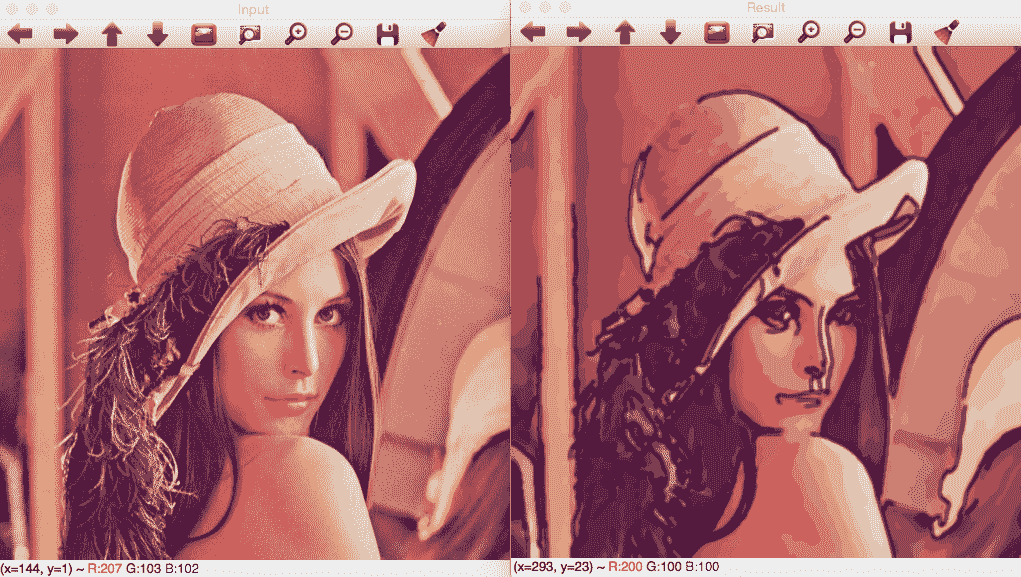# 四、深入研究直方图和过滤器

• 直方图和直方图均衡化
• 查找表
• 模糊和中间模糊
• 精明过滤器
• 图像颜色均衡
• 了解图像类型之间的转换

• 生成 CMake 脚本文件
• 创建图形用户界面
• 计算和绘制直方图
• 直方图均衡
• Lomography 相机效果
• 卡通化效果

# 生成 CMake 脚本文件

cmake_minimum_required (VERSION 3.0)

PROJECT(Chapter4_Phototool)

set (CMAKE_CXX_STANDARD 11)

# Requires OpenCV
FIND_PACKAGE( OpenCV 4.0.0 REQUIRED )
MESSAGE("OpenCV version : ${OpenCV_VERSION}") include_directories(${OpenCV_INCLUDE_DIRS})
link_directories(${OpenCV_LIB_DIR}) ADD_EXECUTABLE(${PROJECT_NAME} main.cpp)
TARGET_LINK_LIBRARIES(${PROJECT_NAME}${OpenCV_LIBS})




include_directories(${OpenCV_INCLUDE_DIRS}) link_directories(${OpenCV_LIB_DIR})


ADD_EXECUTABLE(${PROJECT_NAME} main.cpp) TARGET_LINK_LIBRARIES(${PROJECT_NAME} \${OpenCV_LIBS})


# 创建图形用户界面

• 显示直方图
• 均衡化直方图
• 光照相效果
• 卡通化效果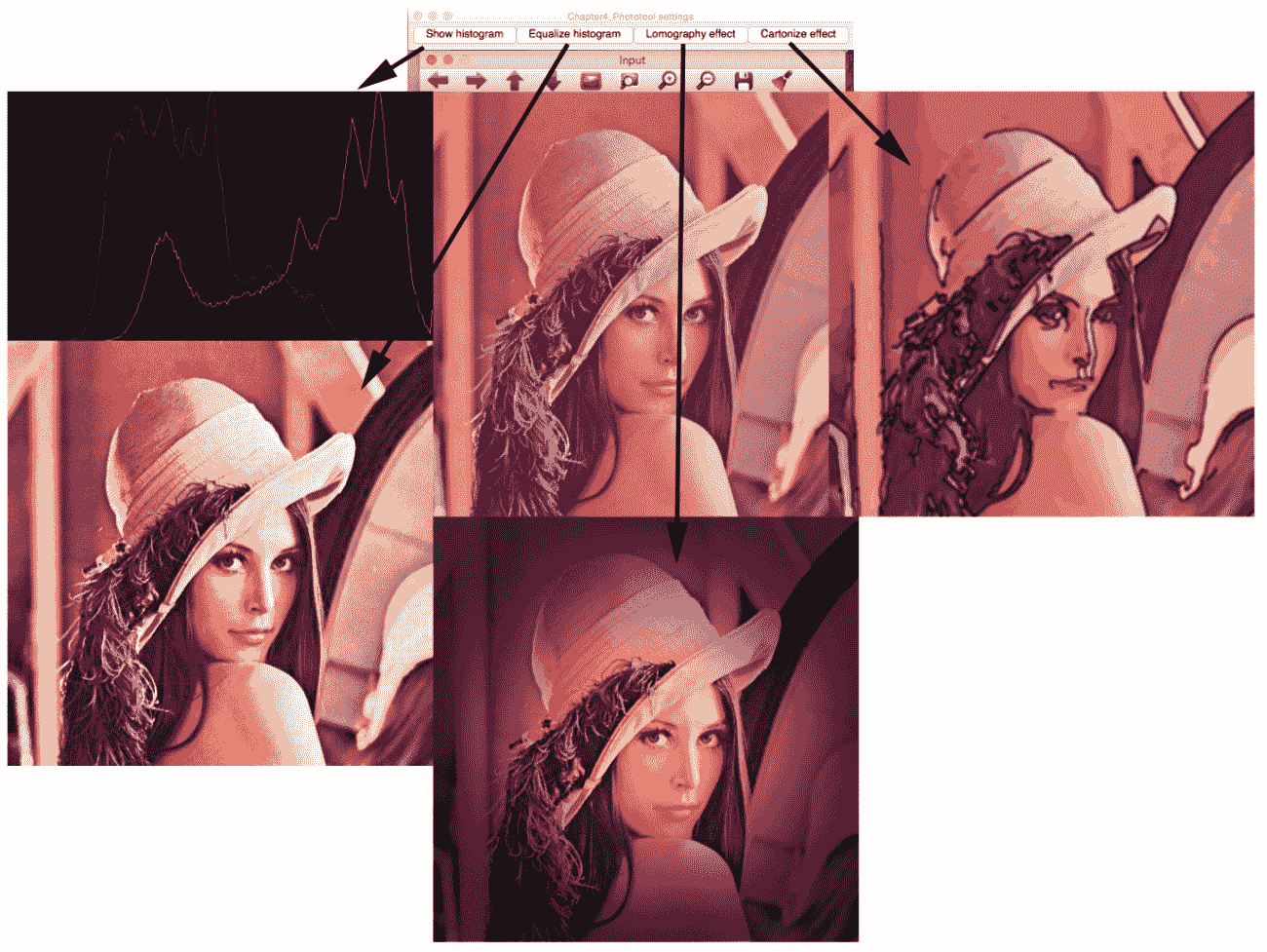// OpenCV includes
#include "opencv2/core/utility.hpp"
#include "opencv2/imgproc.hpp"
#include "opencv2/highgui.hpp"
using namespace cv;
// OpenCV command line parser functions
// Keys accepted by command line parser
const char* keys =
{
"{help h usage ? | | print this message}"
"{@image | | Image to process}"
};


Main 函数从命令行解析器变量开始；接下来，我们设置关于指令并打印帮助消息。 该行设置最终可执行文件的帮助指令：

int main(int argc, const char** argv)
{
CommandLineParser parser(argc, argv, keys);
//If requires help show
if (parser.has("help"))
{
parser.printMessage();
return 0;
}


String imgFile= parser.get<String>(0);

// Check if params are correctly parsed in his variables
if (!parser.check())
{
parser.printErrors();
return 0;
}


// Load image to process

// Create window
namedWindow("Input");


// Create UI buttons
createButton("Show histogram", showHistoCallback, NULL, QT_PUSH_BUTTON, 0);
createButton("Equalize histogram", equalizeCallback, NULL, QT_PUSH_BUTTON, 0);
createButton("Lomography effect", lomoCallback, NULL, QT_PUSH_BUTTON, 0);
createButton("Cartoonize effect", cartoonCallback, NULL, QT_PUSH_BUTTON, 0);


// Show image
imshow("Input", img);

waitKey(0);
return 0;


# 绘制直方图

void showHistoCallback(int state, void* userData)
{
// Separate image in BRG
vector<Mat> bgr;
split(img, bgr);

// Create the histogram for 256 bins
// The number of possibles values [0..255]
int numbins= 256;

/// Set the ranges for B,G,R last is not included
float range[] = { 0, 256 } ;
const float* histRange = { range };

Mat b_hist, g_hist, r_hist;

calcHist(&bgr, 1, 0, Mat(), b_hist, 1, &numbins, &histRange);
calcHist(&bgr, 1, 0, Mat(), g_hist, 1, &numbins, &histRange);
calcHist(&bgr, 1, 0, Mat(), r_hist, 1, &numbins, &histRange);

// Draw the histogram
// We go to draw lines for each channel
int width= 512;
int height= 300;
// Create image with gray base
Mat histImage(height, width, CV_8UC3, Scalar(20,20,20));

// Normalize the histograms to height of image
normalize(b_hist, b_hist, 0, height, NORM_MINMAX);
normalize(g_hist, g_hist, 0, height, NORM_MINMAX);
normalize(r_hist, r_hist, 0, height, NORM_MINMAX);

int binStep= cvRound((float)width/(float)numbins);
for(int i=1; i< numbins; i++)
{
line(histImage,
Point( binStep*(i-1), height-cvRound(b_hist.at<float>(i-1) )),
Point( binStep*(i), height-cvRound(b_hist.at<float>(i) )),
Scalar(255,0,0)
);
line(histImage,
Point(binStep*(i-1), height-cvRound(g_hist.at<float>(i-1))),
Point(binStep*(i), height-cvRound(g_hist.at<float>(i))),
Scalar(0,255,0)
);
line(histImage,
Point(binStep*(i-1), height-cvRound(r_hist.at<float>(i-1))),
Point(binStep*(i), height-cvRound(r_hist.at<float>(i))),
Scalar(0,0,255)
);
}

imshow("Histogram", histImage);

}


// Separate image in BRG
vector<Mat> bgr;
split(img, bgr);


int numbins= 256;


/// Set the ranges for B,G,R
float range[] = {0, 256} ;
const float* histRange = {range};

Mat b_hist, g_hist, r_hist;


• 输入图像：在我们的示例中，我们使用存储在bgr向量中的一个图像通道
• 输入中用于计算直方图的图像数量：在我们的示例中，我们只使用1图像
• 用于计算直方图的数字通道维度：我们在本例中使用[T0
• 可选的掩码矩阵。
• 用于存储计算出的直方图的变量。
• 直方图维度：这是图像(这里是灰色平面)取值的空间维度，在我们的示例中为1
• 要计算的条柱数量：在我们的示例中为256个条柱，每个像素值一个
• 输入变量的范围：在我们的例子中，可能的像素值从0255

calcHist(&bgr, 1, 0, Mat(), b_hist, 1, &numbins, &histRange );
calcHist(&bgr, 1, 0, Mat(), g_hist, 1, &numbins, &histRange );
calcHist(&bgr, 1, 0, Mat(), r_hist, 1, &numbins, &histRange );


// Draw the histogram
// We go to draw lines for each channel
int width= 512;
int height= 300;
// Create image with gray base
Mat histImage(height, width, CV_8UC3, Scalar(20,20,20));


// Normalize the histograms to height of image
normalize(b_hist, b_hist, 0, height, NORM_MINMAX);
normalize(g_hist, g_hist, 0, height, NORM_MINMAX);
normalize(r_hist, r_hist, 0, height, NORM_MINMAX);


int binStep= cvRound((float)width/(float)numbins);
for(int i=1; i< numbins; i++)
{
line(histImage,
Point(binStep*(i-1), height-cvRound(b_hist.at<float>(i-1))),
Point(binStep*(i), height-cvRound(b_hist.at<float>(i))),
Scalar(255,0,0)
);
line(histImage,
Point(binStep*(i-1), height-cvRound(g_hist.at<float>(i-1))),
Point( binStep*(i), height-cvRound(g_hist.at<float>(i))),
Scalar(0,255,0)
);
line(histImage,
Point(binStep*(i-1), height-cvRound(r_hist.at<float>(i-1))),
Point( binStep*(i), height-cvRound(r_hist.at<float>(i))),
Scalar(0,0,255)
);
}


    imshow("Histogram", histImage);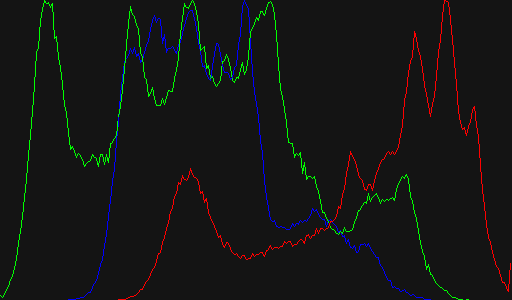# 图像色彩均衡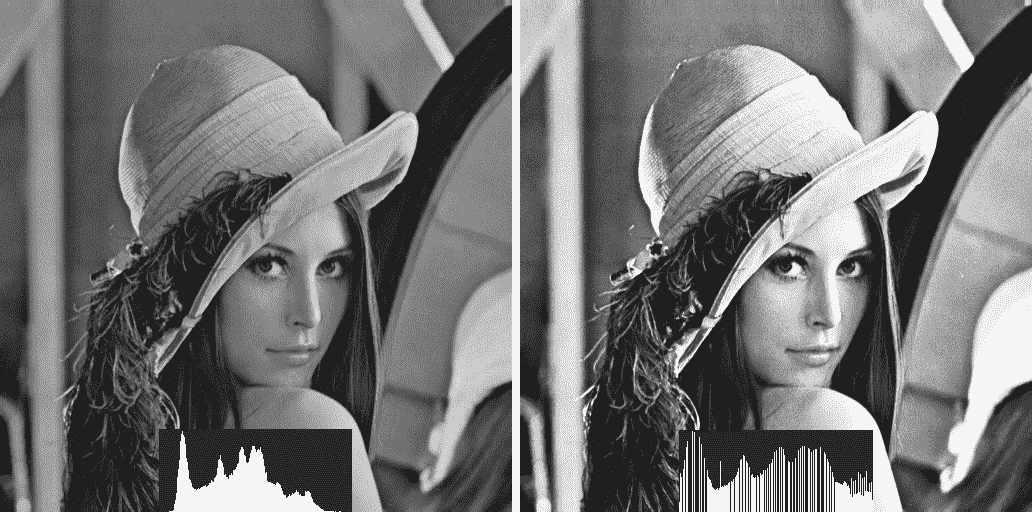void equalizeCallback(int state, void* userData)
{
Mat result;
// Convert BGR image to YCbCr
Mat ycrcb;
cvtColor(img, ycrcb, COLOR_BGR2YCrCb);

// Split image into channels
vector<Mat> channels;
split(ycrcb, channels);

// Equalize the Y channel only
equalizeHist(channels, channels);

// Merge the result channels
merge(channels, ycrcb);

// Convert color ycrcb to BGR
cvtColor(ycrcb, result, COLOR_YCrCb2BGR);

// Show image
imshow("Equalized", result);
}


1.使用cvtColor函数将bgr图像转换或输入为YCrCb

Mat result;
// Convert BGR image to YCbCr
Mat ycrcb;
cvtColor(img, ycrcb, COLOR_BGR2YCrCb);


2.将YCrCb镜像拆分成不同的通道矩阵：

// Split image into channels
vector<Mat> channels;
split(ycrcb, channels);


3.使用只有两个参数(输入和输出矩阵)的equalizeHist函数，仅均衡 Y 通道中的直方图：

// Equalize the Y channel only
equalizeHist(channels, channels);


4.合并生成的通道，并将其转换为BGR格式，向用户显示结果：

// Merge the result channels
merge(channels, ycrcb);

// Convert color ycrcb to BGR
cvtColor(ycrcb, result, COLOR_YCrCb2BGR);

// Show image
imshow("Equalized", result);# 光照相效果

void lomoCallback(int state, void* userData)
{
Mat result;

const double exponential_e = std::exp(1.0);
// Create Look-up table for color curve effect
Mat lut(1, 256, CV_8UC1);
for (int i=0; i<256; i++)
{
float x= (float)i/256.0;
lut.at<uchar>(i)= cvRound( 256 * (1/(1 + pow(exponential_e, -((x-0.5)/0.1)) )) );
}

// Split the image channels and apply curve transform only to red channel
vector<Mat> bgr;
split(img, bgr);
LUT(bgr, lut, bgr);
// merge result
merge(bgr, result);

// Create image for halo dark
Mat halo(img.rows, img.cols, CV_32FC3, Scalar(0.3,0.3,0.3) );
// Create circle
circle(halo, Point(img.cols/2, img.rows/2), img.cols/3, Scalar(1,1,1), -1);
blur(halo, halo, Size(img.cols/3, img.cols/3));

// Convert the result to float to allow multiply by 1 factor
Mat resultf;
result.convertTo(resultf, CV_32FC3);

// Multiply our result with halo
multiply(resultf, halo, resultf);

// convert to 8 bits
resultf.convertTo(result, CV_8UC3);

// show result
imshow("Lomography", result);
}


1. 通过使用查找表将曲线应用于红色通道来实现颜色操纵效果
2. 通过在图像上应用深色光晕来实现复古效果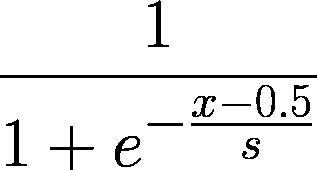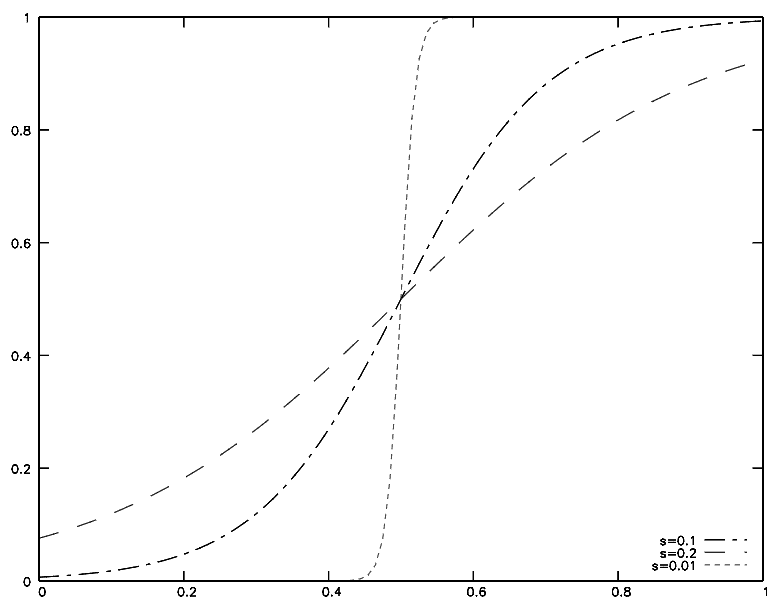const double exponential_e = std::exp(1.0);
// Create look-up table for color curve effect
Mat lut(1, 256, CV_8UC1);
Uchar* plut= lut.data;
for (int i=0; i<256; i++)
{
double x= (double)i/256.0;
plut[i]= cvRound( 256.0 * (1.0/(1.0 + pow(exponential_e, -((x-0.5)/0.1)) )) );
}


// Split the image channels and apply curve transform only to red channel
vector<Mat> bgr;
split(img, bgr);


• 输入图像
• 查找表矩阵
• 输出图像

LUT(bgr, lut, bgr);


// merge result
merge(bgr, result);


 // Create image for halo dark
Mat halo(img.rows, img.cols, CV_32FC3, Scalar(0.3,0.3,0.3));
// Create circle
circle(halo, Point(img.cols/2, img.rows/2), img.cols/3, Scalar(1,1,1), -1);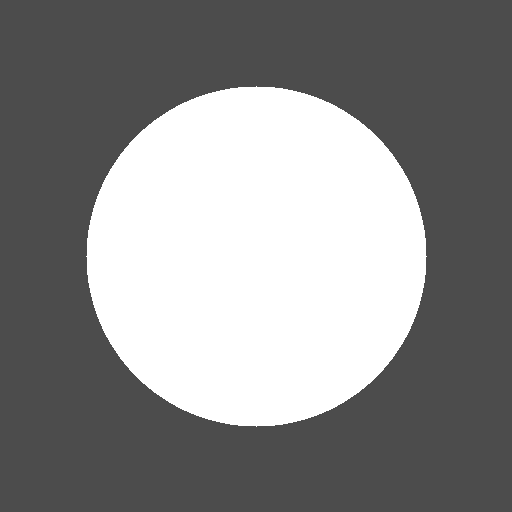blur(halo, halo, Size(img.cols/3, img.cols/3));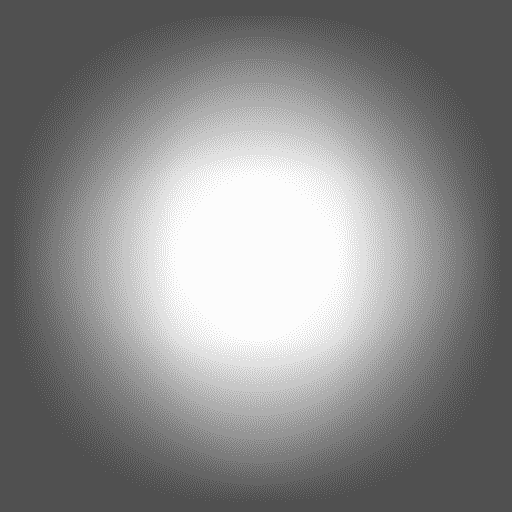// Convert the result to float to allow multiply by 1 factor
Mat resultf;
result.convertTo(resultf, CV_32FC3);


// Multiply our result with halo
multiply(resultf, halo, resultf);


// convert to 8 bits
resultf.convertTo(result, CV_8UC3);

// show result
imshow("Lomograpy", result);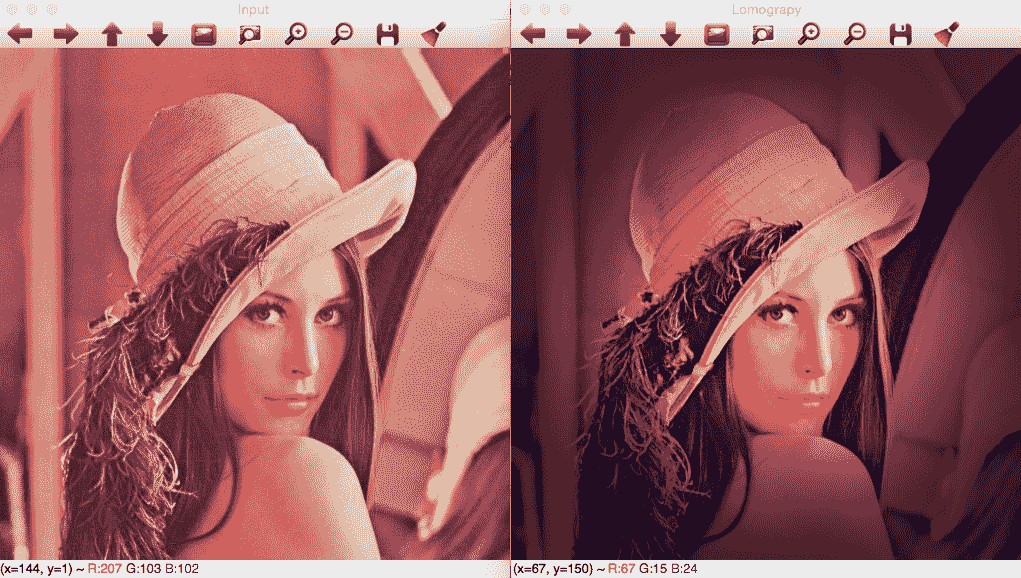# 卡通化效果

cartoonCallback函数定义此效果，其代码如下：

void cartoonCallback(int state, void* userData)
{
/** EDGES **/
// Apply median filter to remove possible noise
Mat imgMedian;
medianBlur(img, imgMedian, 7);

// Detect edges with canny
Mat imgCanny;
Canny(imgMedian, imgCanny, 50, 150);

// Dilate the edges
Mat kernel= getStructuringElement(MORPH_RECT, Size(2,2));
dilate(imgCanny, imgCanny, kernel);

// Scale edges values to 1 and invert values
imgCanny= imgCanny/255;
imgCanny= 1-imgCanny;

// Use float values to allow multiply between 0 and 1
Mat imgCannyf;
imgCanny.convertTo(imgCannyf, CV_32FC3);

// Blur the edgest to do smooth effect
blur(imgCannyf, imgCannyf, Size(5,5));

/** COLOR **/
// Apply bilateral filter to homogenizes color
Mat imgBF;
bilateralFilter(img, imgBF, 9, 150.0, 150.0);

// truncate colors
Mat result= imgBF/25;
result= result*25;

/** MERGES COLOR + EDGES **/
// Create a 3 channles for edges
Mat imgCanny3c;
Mat cannyChannels[]={ imgCannyf, imgCannyf, imgCannyf};
merge(cannyChannels, 3, imgCanny3c);

// Convert color result to float
Mat resultf;
result.convertTo(resultf, CV_32FC3);

// Multiply color and edges matrices
multiply(resultf, imgCanny3c, resultf);

// convert to 8 bits color
resultf.convertTo(result, CV_8UC3);

// Show image
imshow("Result", result);

}


Mat imgMedian;
medianBlur(img, imgMedian, 7);


// Detect edges with canny
Mat imgCanny;
Canny(imgMedian, imgCanny, 50, 150);


Canny过滤器接受以下参数：

• 输入图像
• 输出图像
• 第一阈值
• 第二阈值
• 索贝尔大小孔径
• 布尔值，指示我们是否需要使用更精确的图像渐变幅度

// Dilate the edges
Mat kernel= getStructuringElement(MORPH_RECT, Size(2,2));
dilate(imgCanny, imgCanny, kernel);


// Scale edges values to 1 and invert values
imgCanny= imgCanny/255;
imgCanny= 1-imgCanny;


// Use float values to allow multiply between 0 and 1
Mat imgCannyf;
imgCanny.convertTo(imgCannyf, CV_32FC3);


// Blur the edgest to do smooth effect
blur(imgCannyf, imgCannyf, Size(5,5));


// Apply bilateral filter to homogenizes color
Mat imgBF;
bilateralFilter(img, imgBF, 9, 150.0, 150.0);


bilateral滤波器是一种在保持边缘的同时降低图像噪声的滤波器。 有了适当的参数，我们将在后面讨论，我们可以得到卡通效果。

bilateral过滤器的参数如下：

• 输入图像
• 输出图像
• 像素邻域的直径；如果设置为负值，则根据 sigma 空间值计算

• 西格玛颜色值

• 西格玛坐标空间

With a diameter greater than five, the bilateral filter starts to become slow. With sigma values greater than 150, a cartoonish effect appears.

// truncate colors
Mat result= imgBF/25;
result= result*25;


// Create a 3 channles for edges
Mat imgCanny3c;
Mat cannyChannels[]={ imgCannyf, imgCannyf, imgCannyf};
merge(cannyChannels, 3, imgCanny3c);


// Convert color result to float
Mat resultf;
result.convertTo(resultf, CV_32FC3);

// Multiply color and edges matrices
multiply(resultf, imgCanny3c, resultf);


// convert to 8 bits color
resultf.convertTo(result, CV_8UC3);

// Show image
imshow("Result", result);